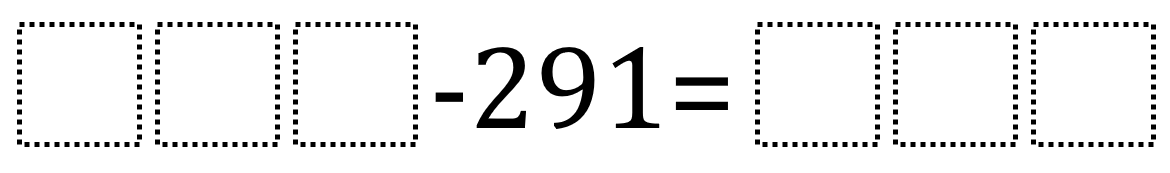# Subtracting 3-Digit Numbers 1

Directions: Using the digits 1 to 9 at most one time each, place a digit in each box to make two different pairs of three-digit numbers that form a true number sentence. You may reuse all the digits each difference.### Hint

How can we determine which digits would be poor choices to place?

There are many possible answers including 874 – 291 = 583 and 587 – 291 = 296. To be clear, the 291 in the number being subtracted to not count as being used.

Source: Robert Kaplinsky

## Multiply and Divide Within A Hundred 1

Directions: Using the digits 2 to 9 at most one time each, place a digit …

1.I am working on this with my students right now and they were curious why you decided to subtract 291 of all the 3 digit numbers. Thanks!

•Robert Kaplinsky

Gosh, that’s a great question. I’m trying to remember why I picked that number. I know that some numbers would be trivial. For example subtracting 100 would not even be interesting. I think I wanted to do something that would almost certainly require there to be some kind of borrowing or regrouping.

2.354 – 291 = 352

543 – 291 = 352